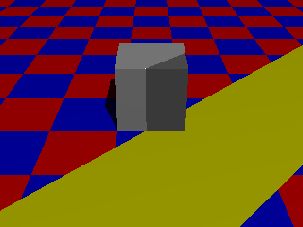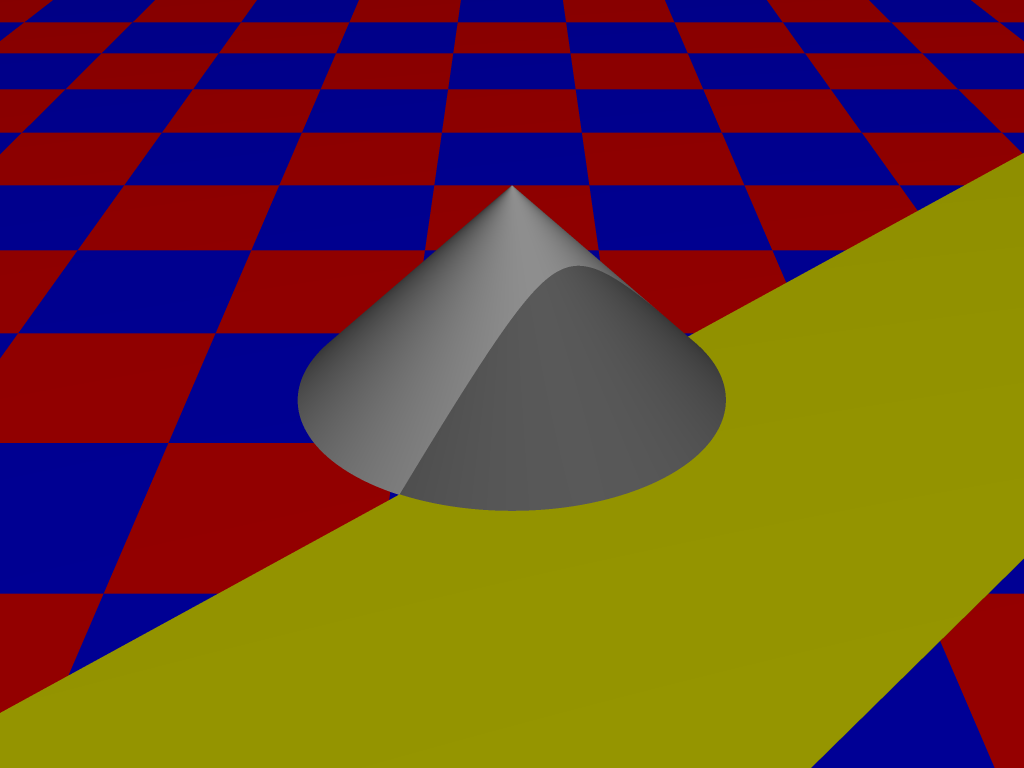2.6.3 Gupta-Sproull Anti-aliasing Algorithm

cf. [FvDFH90, 3.17.4]

This algorithm weights the intersecting area, by considering a cone with radius 1 (hence a line with slope 1 intersects 3 vertical pixels) and take the volume of the part of this cone lying above the rectangle representing the line. To speed things up, we need not calculate these volumina during runtime but can use a fixed pre-calculated table of intensities depending on the various distances to the center. We can use the scan-conversion algorithm to determine the approximation points, but set the intensity levels of then and the two vertically neighboring pixels according to their distance.Andreas Kriegl 2003-07-23